# DAV Class 5 Maths Chapter 7 Worksheet 11 Solutions

The DAV Maths Book Class 5 Solutions and DAV Class 5 Maths Chapter 7 Worksheet 11 Solutions of Multiplication and Division of Decimal Numbers offer comprehensive answers to textbook questions.

## DAV Class 5 Maths Ch 7 Worksheet 11 Solutions

Question 1.
Convert the following fractions into a decimal number.
(a) $$\frac{3}{4}$$
Solution:
$$\frac{3}{4}$$ = 3 ÷ 4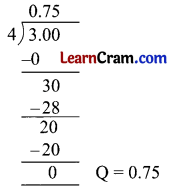(b) $$\frac{7}{8}$$
Solution:
$$\frac{7}{8}$$ = 7 ÷ 8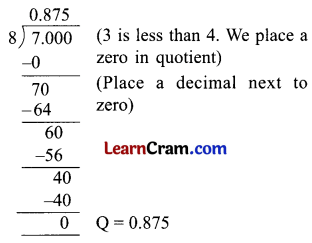(c) $$\frac{1}{20}$$
Solution:
$$\frac{1}{20}$$ = 1 ÷ 20(d) $$\frac{8}{25}$$
Solution:
$$\frac{8}{25}$$ = 8 ÷ 25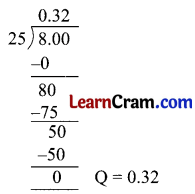(e) $$\frac{18}{90}$$
Solution:
$$\frac{18}{90}$$ = 18 ÷ 90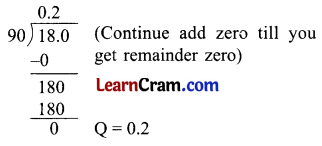(f) $$\frac{12}{15}$$
Solution:
$$\frac{12}{15}$$ = 12 ÷ 15(g) $$\frac{11}{20}$$
Solution:
$$\frac{11}{20}$$ = 11 ÷ 20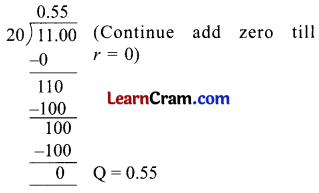(h) $$\frac{31}{50}$$
Solution:
$$\frac{31}{50}$$ = 31 ÷ 50Question 2.
Convert the following into a decimal number.
(a) 1$$\frac{1}{2}$$
Solution: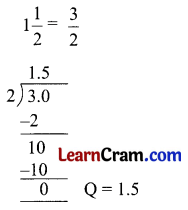(b) 5$$\frac{1}{5}$$
Solution: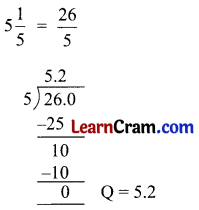(c) 16$$\frac{1}{5}$$
Solution: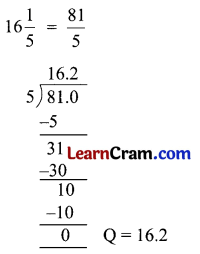(d) 4$$\frac{1}{20}$$
Solution: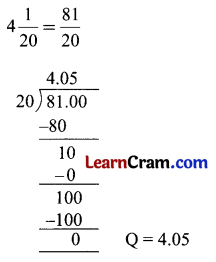(e) 4$$\frac{1}{4}$$
Solution:(f) 4$$\frac{3}{8}$$
Solution: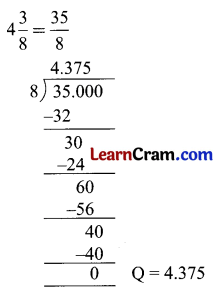(g) 1$$\frac{2}{25}$$
Solution: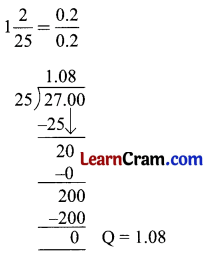(h) 1$$\frac{3}{4}$$
Solution:Word Problems

Example 1.
Convert $$\frac{2}{5}$$ into a decimal number.
Solution:
$$\frac{2}{5}$$ = 2 ÷ 5 (Fraction as division sum)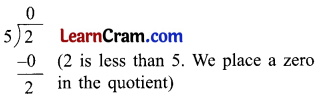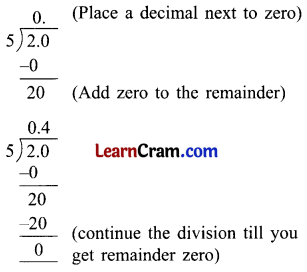Example 2.
Convert $$\frac{33}{8}$$ into a decimal number.
Solution:Continue adding zeroes to the remainder and divide till you get the remainder zero.